• No products in the cart.

# CURRENT ELECTRICITY A-LEVEL

##### This Unit is about Current Electricity and how it works.

CURRENT ELECTRICITY

Conduction of electricity in metals

Metals have electrons that are loosely bound to the nuclei and are free to wander randomly through the metal from one point to the other. When the p.d is applied across the ends of the metal, an electric filed is set up. Electrons are accelerated by the field and so they gain kinetic energy. The accelerated electrons collide with atoms vibrating about a fixed mean position.

They give out some of their energy to the atoms. The amplitude of vibrations of atoms is then increased and the temperature of the atoms rises.

Electrons are again accelerated by the field and again  give up some of their energy on collision with the atoms of the metal. The overall acceleration of electrons is zero hence they acquire a constant average drift velocity, in the direction from the negative to the positive terminals of the battery. It is this drift of electrons that constitute an electric  current.

In general, current flow in metals is due to movement of electrons (negative charge). In gases and electrolytes, both positive and negative charges are involved.

Unit of charge: the coulomb (C)

A coulomb is the quantity of electric charge carried past a given point in a circuit when a steady current of  one ampere flows for one second.

i.e. Charge, Q = current, I x time, t

Q = It

1C = 1As

Drift velocity

Consider a conductor of length l, cross sectional  area A, having n electrons per unit volume, each carrying a charge e.Volume of  conductor = Al

Number of electrons  in the conductor = nAl

Total charge, Q = nAle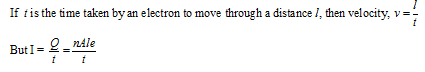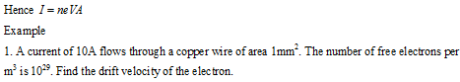1. A metal wire contains 5×1022 electrons per cm3 and has cross sectional area off 1mm2. If the electrons move along the wire with a mean drift velocity of 1mms-1, Calculate:

(i) current density

(ii) current in the wire.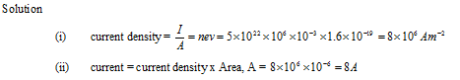THIS VIDEO EXPLAINS MORE ABOUT CURRENT ELECTRICITY

Ohm’s Law

Under constant physical conditions, the potential difference across a conductor is proportional to the current through it.The constant is the resistance R of the conductor.

Hence V= IV

The unit of resistance  is the ohm (W)

The ohm is the resistance of a conductor in which a current of one ampere flows when a p.d of one volt is applied across it.

A graph of I against V for a homogeneous conductor.Graphs of I against V for non- Ohmic conductors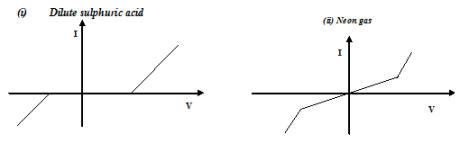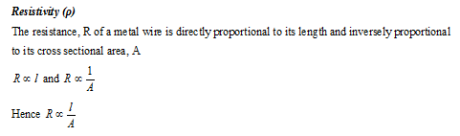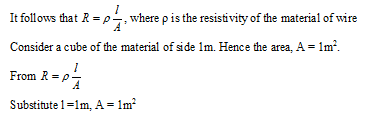THIS VIDEO IS ABOUT OHM’S LAW

Electromotive force, emf (e) and internal resistance (r )   of the cell

Emf of a source is the energy converted into electrical energy when 1C of charge passes through it.

Or it is the ratio of electrical power the source generates to the current which it delivers.

Internal resistance is the effective resistance of the source which accounts for the energy losses in it. Internal resistance behaves as if it is a resistor in series with the battery.

Hence

Energy supplied per coulomb by cell = (energy changed per coulomb by external circuit)

+(energy wasted per coulomb on internal resistance )A graph of V against I is shown below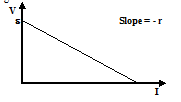Also V = IRPower out put and efficiencyHence a graph of h against Load resistance R  is shown below: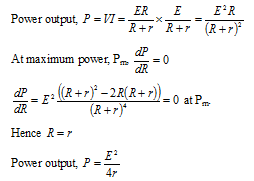As R tends to zero, P tends to zero

As R tends to ¥, P tends to zero.

A graph of power out put P against load resistance R is shown below.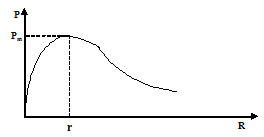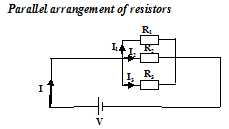For parallel

• same p.d across each resistor
• total current, I is equal to sum of Current through each resistor.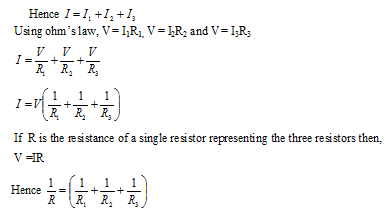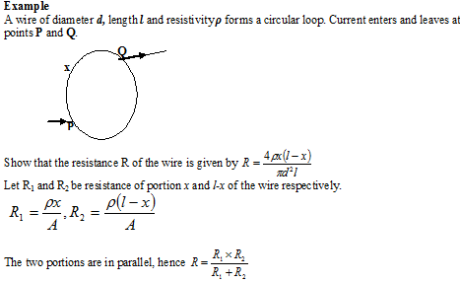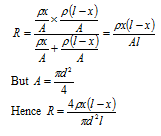G is centre zero galvanometer

R1 is unknown resistor

R2 is standard resistor (known)

R3 and R4 are variable resistors where resistance can be read e.g. resistance box.

As R3 and R4 are varied, the potential at B and D change.

• If the potential at B, VB is greater than that at D, VD, then current ig flows from B to D giving a deflection on one side of the galvanometer.
• If VD > VB, ig flows from D to B, galvanometer flows in the opposite direction.
• If VD = VB, ig = 0, galvanometer indicates zero deflection. In this case the Wheat stone bridge is said to be balanced.

Therefore to balance the bridge, R3 and R4 are varied until the galvanometer indicates zero deflection.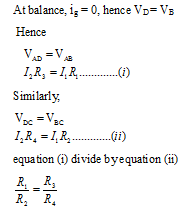The above equation is the condition for a Wheatstone bridge to balance.

Simple metre bridge

It is a form of a Wheatstone bridge with a resistance wire of uniform cross section area mounted on a metre rule. It is used to measure resistance, resistivity and temperature coefficient of resistance.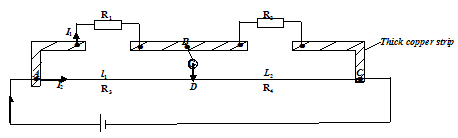Condition for a metre bridge to balance.

At balance, ig = 0, hence VD = VB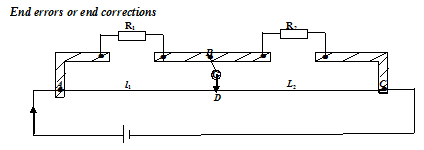Owing to imperfect electrical contacts at A and C, contacts have small resistance which affect the accuracy of the results. But the effect on the accuracy becomes less significant if the balance point is near the 50cm mark.

For accuracy, the contact resistances have to be considered.  The contact resistances at A and C are equivalent to extra lengths e1 and e2 of the slide wire. e1 and e2 are called end corrections or end errors.

Hence end errors have to be added to the balance lengths to account for the resistances at the contacts at the ends of the slide wire.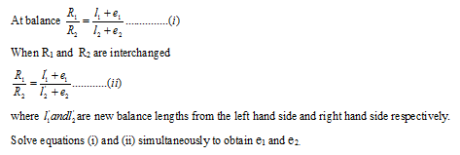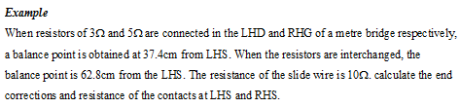A specimen wire of known length x is connected in the left hand gap of a metre bridge with a standard resistor, Rs in the right hand gap of the bridge. The slider is moved along AC until the galvanometer indicates zero deflection. At this point  the metre bridge is balanced. The balance length l from the left hand side of the metre bridge is measured. The experiment is  repeated for different values of x and the corresponding balance lengths l are measured and recorded.

Theory of experiment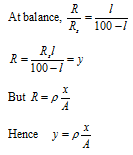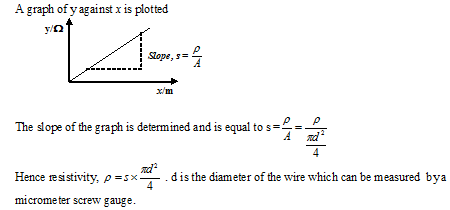Temperature coefficient (α ) of a metal wire

The mean temperature coefficient of a material  can be defined as the fractional increase in resistance at 0°C for every °C increase in temperature.The specimen wire is made into a coil and immersed in water basin whose temperature θ°C is varied and measured with a thermometer placed in the water. The coil is connected to the left hand gap of the bridge and a standard resistor Rto the right hand gap.

At each temperature, θ°C, the balance length l from the left hand side id measured and recorded.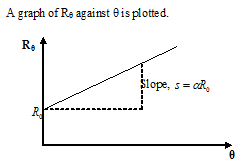Examples

When a coil x is connected across the Left hand gap of a metre bridge and heated to a temperature of 30°C, the balance point is found to be 51.5°C from the left hand side of the slide wire. when the temperature is raised to 100°C, the balance point is 54.6cm from the left hand side. Find the temperature coefficient of resistance of x.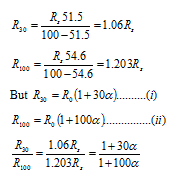When a coil x is connected across the Left hand gap of a metre bridge and heated to a temperature of 30°C, the balance point is found to be 51.5°C from the left hand side of the slide wire. when the temperature is raised to 100°C, the balance point is 54.6cm from the left hand side. Find the temperature coefficient of resistance of x.

When a coil x is connected across the Left hand gap of a metre bridge and heated to a temperature of 30°C, the balance point is found to be 51.5°C from the left hand side of the slide wire. when the temperature is raised to 100°C, the balance point is 54.6cm from the left hand side. Find the temperature coefficient of resistance of x.